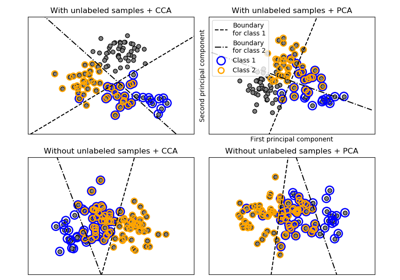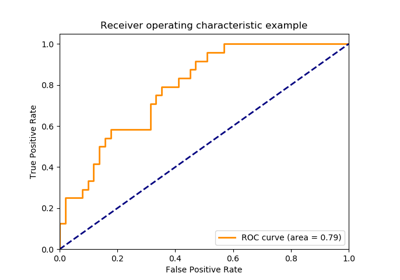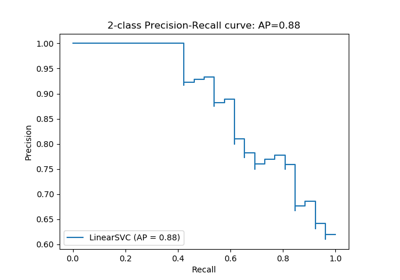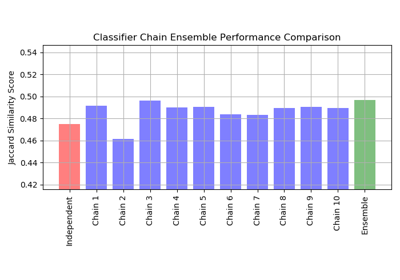# sklearn.multiclass.OneVsRestClassifier¶

class sklearn.multiclass.OneVsRestClassifier(estimator, n_jobs=None)[source]

One-vs-the-rest (OvR) multiclass/multilabel strategy

Also known as one-vs-all, this strategy consists in fitting one classifier per class. For each classifier, the class is fitted against all the other classes. In addition to its computational efficiency (only n_classes classifiers are needed), one advantage of this approach is its interpretability. Since each class is represented by one and one classifier only, it is possible to gain knowledge about the class by inspecting its corresponding classifier. This is the most commonly used strategy for multiclass classification and is a fair default choice.

This strategy can also be used for multilabel learning, where a classifier is used to predict multiple labels for instance, by fitting on a 2-d matrix in which cell [i, j] is 1 if sample i has label j and 0 otherwise.

In the multilabel learning literature, OvR is also known as the binary relevance method.

Read more in the User Guide.

Parameters
estimatorestimator object

An estimator object implementing fit and one of decision_function or predict_proba.

n_jobsint or None, optional (default=None)

The number of jobs to use for the computation. None means 1 unless in a joblib.parallel_backend context. -1 means using all processors. See Glossary for more details.

Attributes
estimators_list of n_classes estimators

Estimators used for predictions.

classes_array, shape = [n_classes]

Class labels.

n_classes_int

Number of classes.

label_binarizer_LabelBinarizer object

Object used to transform multiclass labels to binary labels and vice-versa.

multilabel_boolean

Whether this is a multilabel classifier

Examples

>>> import numpy as np
>>> from sklearn.multiclass import OneVsRestClassifier
>>> from sklearn.svm import SVC
>>> X = np.array([
...     [10, 10],
...     [8, 10],
...     [-5, 5.5],
...     [-5.4, 5.5],
...     [-20, -20],
...     [-15, -20]
... ])
>>> y = np.array([0, 0, 1, 1, 2, 2])
>>> clf = OneVsRestClassifier(SVC()).fit(X, y)
>>> clf.predict([[-19, -20], [9, 9], [-5, 5]])
array([2, 0, 1])


Methods

 decision_function(self, X) Returns the distance of each sample from the decision boundary for each class. fit(self, X, y) Fit underlying estimators. get_params(self[, deep]) Get parameters for this estimator. partial_fit(self, X, y[, classes]) Partially fit underlying estimators predict(self, X) Predict multi-class targets using underlying estimators. predict_proba(self, X) Probability estimates. score(self, X, y[, sample_weight]) Return the mean accuracy on the given test data and labels. set_params(self, \*\*params) Set the parameters of this estimator.
__init__(self, estimator, n_jobs=None)[source]

Initialize self. See help(type(self)) for accurate signature.

decision_function(self, X)[source]

Returns the distance of each sample from the decision boundary for each class. This can only be used with estimators which implement the decision_function method.

Parameters
Xarray-like of shape (n_samples, n_features)
Returns
Tarray-like of shape (n_samples, n_classes)
fit(self, X, y)[source]

Fit underlying estimators.

Parameters
X(sparse) array-like of shape (n_samples, n_features)

Data.

y(sparse) array-like of shape (n_samples,) or (n_samples, n_classes)

Multi-class targets. An indicator matrix turns on multilabel classification.

Returns
self
get_params(self, deep=True)[source]

Get parameters for this estimator.

Parameters
deepbool, default=True

If True, will return the parameters for this estimator and contained subobjects that are estimators.

Returns
paramsmapping of string to any

Parameter names mapped to their values.

property multilabel_

Whether this is a multilabel classifier

partial_fit(self, X, y, classes=None)[source]

Partially fit underlying estimators

Should be used when memory is inefficient to train all data. Chunks of data can be passed in several iteration.

Parameters
X(sparse) array-like of shape (n_samples, n_features)

Data.

y(sparse) array-like of shape (n_samples,) or (n_samples, n_classes)

Multi-class targets. An indicator matrix turns on multilabel classification.

classesarray, shape (n_classes, )

Classes across all calls to partial_fit. Can be obtained via np.unique(y_all), where y_all is the target vector of the entire dataset. This argument is only required in the first call of partial_fit and can be omitted in the subsequent calls.

Returns
self
predict(self, X)[source]

Predict multi-class targets using underlying estimators.

Parameters
X(sparse) array-like of shape (n_samples, n_features)

Data.

Returns
y(sparse) array-like of shape (n_samples,) or (n_samples, n_classes)

Predicted multi-class targets.

predict_proba(self, X)[source]

Probability estimates.

The returned estimates for all classes are ordered by label of classes.

Note that in the multilabel case, each sample can have any number of labels. This returns the marginal probability that the given sample has the label in question. For example, it is entirely consistent that two labels both have a 90% probability of applying to a given sample.

In the single label multiclass case, the rows of the returned matrix sum to 1.

Parameters
Xarray-like of shape (n_samples, n_features)
Returns
T(sparse) array-like of shape (n_samples, n_classes)

Returns the probability of the sample for each class in the model, where classes are ordered as they are in self.classes_.

score(self, X, y, sample_weight=None)[source]

Return the mean accuracy on the given test data and labels.

In multi-label classification, this is the subset accuracy which is a harsh metric since you require for each sample that each label set be correctly predicted.

Parameters
Xarray-like of shape (n_samples, n_features)

Test samples.

yarray-like of shape (n_samples,) or (n_samples, n_outputs)

True labels for X.

sample_weightarray-like of shape (n_samples,), default=None

Sample weights.

Returns
scorefloat

Mean accuracy of self.predict(X) wrt. y.

set_params(self, **params)[source]

Set the parameters of this estimator.

The method works on simple estimators as well as on nested objects (such as pipelines). The latter have parameters of the form <component>__<parameter> so that it’s possible to update each component of a nested object.

Parameters
**paramsdict

Estimator parameters.

Returns
selfobject

Estimator instance.

## Examples using sklearn.multiclass.OneVsRestClassifier¶